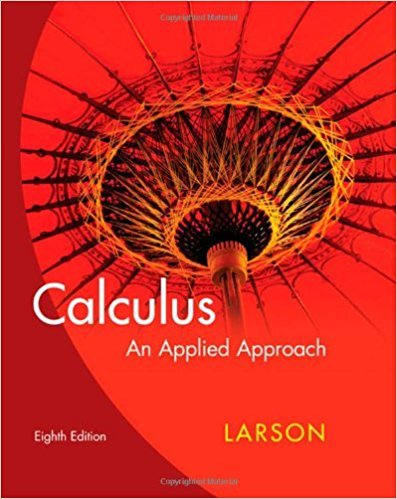×
×

# Solutions for Chapter 2.4: The Product and Quotient Rules## Full solutions for Calculus: An Applied Approach | 8th Edition

ISBN: 9780618958252Solutions for Chapter 2.4: The Product and Quotient Rules

Solutions for Chapter 2.4
4 5 0 310 Reviews
11
1
##### ISBN: 9780618958252

This textbook survival guide was created for the textbook: Calculus: An Applied Approach , edition: 8. Since 72 problems in chapter 2.4: The Product and Quotient Rules have been answered, more than 22543 students have viewed full step-by-step solutions from this chapter. Calculus: An Applied Approach was written by and is associated to the ISBN: 9780618958252. Chapter 2.4: The Product and Quotient Rules includes 72 full step-by-step solutions. This expansive textbook survival guide covers the following chapters and their solutions.

Key Calculus Terms and definitions covered in this textbook
• Augmented matrix

A matrix that represents a system of equations.

• Cardioid

A limaçon whose polar equation is r = a ± a sin ?, or r = a ± a cos ?, where a > 0.

• Common difference

See Arithmetic sequence.

• Focus, foci

See Ellipse, Hyperbola, Parabola.

• Graph of a function ƒ

The set of all points in the coordinate plane corresponding to the pairs (x, ƒ(x)) for x in the domain of ƒ.

• Graph of a polar equation

The set of all points in the polar coordinate system corresponding to the ordered pairs (r,?) that are solutions of the polar equation.

• Infinite discontinuity at x = a

limx:a + x a ƒ(x) = q6 or limx:a - ƒ(x) = q.

• Integrable over [a, b] Lba

ƒ1x2 dx exists.

• Irreducible quadratic over the reals

A quadratic polynomial with real coefficients that cannot be factored using real coefficients.

• Natural numbers

The numbers 1, 2, 3, . . . ,.

• Principal nth root

If bn = a, then b is an nth root of a. If bn = a and a and b have the same sign, b is the principal nth root of a (see Radical), p. 508.

• Rational numbers

Numbers that can be written as a/b, where a and b are integers, and b ? 0.

• Remainder polynomial

See Division algorithm for polynomials.

• Remainder theorem

If a polynomial f(x) is divided by x - c , the remainder is ƒ(c)

• Sample survey

A process for gathering data from a subset of a population, usually through direct questioning.

• Semiminor axis

The distance from the center of an ellipse to a point on the ellipse along a line perpendicular to the major axis.

• Solve a system

To find all solutions of a system.

• Terminal side of an angle

See Angle.

• Union of two sets A and B

The set of all elements that belong to A or B or both.

• Zero factor property

If ab = 0 , then either a = 0 or b = 0.

×